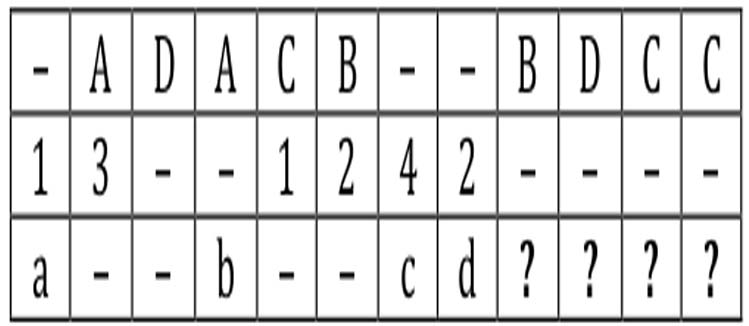Question 18

# In the question below, three incomplete rows of letters/numerals are given which correspond to each other in some way. Find the letters/numerals which come in the vacant places marked by “?”Solution

In Row 1, 2 and Column 5, 6: We can see that corresponding value for letter C and B are 1 and 2 respectively.

In Row 2, 3 and Column 1, 8: We can see that corresponding value for numeric 1 and 2 are 'a' and 'd' respectively.

Hence, we can say that corresponding value in row 3 for letter C and B are 'a' and 'd' respectively.

Hence, the code for B, D, C, C will be of 'd', _, 'a', 'a'. type. Where '_' can be filled by any letter. Hence, option D is the correct answer.

• All Quant Formulas and shortcuts PDF
• 40+ previous papers with solutions PDF